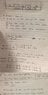# Find transfer function, model and space state of mass spring system

• ashifulk

#### ashifulk

The problem statement:
I have to find the transfer function of a mass spring system. There are two masses in the system and 3 spring. I also have to find the state space representation and the model of the system. I have attached a image of my problem and my work so far. I think I found the model of the system but I'm stuck at the transfer function part. I have two system equations, one for each mass. I'm not sure how I can combine them and get one transfer function.

The attempt at a solution: Please see the attached file. Sorry, I didn't want to type the problem and my solution.

#### Attachments

•homework #1.jpg
19.3 KB · Views: 717

A transfer function relates an output to an input. There's no indication in your attachment of what is considered to be the "input" and what is considered to be the resulting "output". Is there more to the problem statement?

gneill,

I actually didn't understand the problem correctly. The professor mentioned the input forces (f1 and f2) are the output and he said that x1 and x2 are the output. But then yesterday he was going over the this problem and he said you could define the input and output to be anything you wanted (to simplify the problem). So I just assumed f1 and f2 to be the inputs and x1 and x2 to be the outputs.

gneill,

I actually didn't understand the problem correctly. The professor mentioned the input forces (f1 and f2) are the output and he said that x1 and x2 are the output. But then yesterday he was going over the this problem and he said you could define the input and output to be anything you wanted (to simplify the problem). So I just assumed f1 and f2 to be the inputs and x1 and x2 to be the outputs.

If f1 and f2 are inputs, where are they applied? One to each of the masses? Then taking the positions of the masses over time as outputs would make sense.

Are we to assume that the initial conditions have the masses at rest and the springs relaxed?

If f1 and f2 are inputs, where are they applied? One to each of the masses? Then taking the positions of the masses over time as outputs would make sense.

Are we to assume that the initial conditions have the masses at rest and the springs relaxed?

Yes, f1 is applied to M1 and f2 is applied to M2. The initial condition of the masses are at rest and spring relaxed. [f(0) = 0 and f'(0) = 0].

Yes, f1 is applied to M1 and f2 is applied to M2. The initial condition of the masses are at rest and spring relaxed. [f(0) = 0 and f'(0) = 0].

Okay.

Presumably the B's represent friction (damping) due to the masses sliding on a surface? Unlike a dashpot damper, won't these frictions be constant in magnitude regardless of velocity?

I believe the frictional force is a function of velocity, so Fb1 = B1*x1' and Fb2 = B2*x2' where x1' = velocity of M1 and x2' is the velocity of M2.

I believe the frictional force is a function of velocity, so Fb1 = B1*x1' and Fb2 = B2*x2' where x1' = velocity of M1 and x2' is the velocity of M2.

Usually the force due to friction is a function of the normal force and the friction coefficient for the materials. f = μMg for a mass on a horizontal surface, with no velocity dependence other than the direction of the frictional force always opposes that of the velocity.

However, I suppose that as a simplifying assumption for purposes of using Laplace one could take the friction to be -B*x'. This would at least capture the direction-dependence property, although it throws away the constancy property.